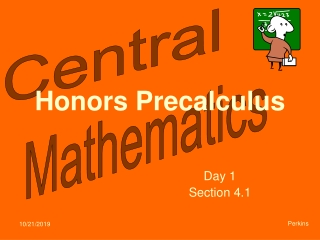DownloadDownload PresentationHonors Precalculus

# Honors Precalculus

Télécharger la présentation## Honors Precalculus

- - - - - - - - - - - - - - - - - - - - - - - - - - - E N D - - - - - - - - - - - - - - - - - - - - - - - - - - -
##### Presentation Transcript

1. Honors Precalculus Day 1 Section 4.1 Perkins

2. One-to-One Functions Will pass both the vertical and horizontal line tests Are either always increasing or always decreasing Inverse functions The inverse of f(x) is written as f -1(x). f(x) and f -1(x) will undo one-another, meaning Only 1-to-1 functions can have inverses (which will require us to limit the domain of those which are not). The domain of f(x) is the same as the range of f -1(x). The range of f(x) is the same as the domain of f -1(x). f(x) and f -1(x) are symmetric about the line y = x. To find f -1(x): Swap x and y. Solve for y.

3. 6 4 2 5 10 -2 -4 -6 Which of these functions are 1-to-1? (not a function)

4. 1. Sketch the graph of the inverse of this 1-to-1 function. Show that these functions are inverses of each other. Method 1: Method 2: graph and look for symmetry about y = x.

5. 3. is a 1-to-1 function. Find its inverse. Swap variables. Solve for y.

6. 4. Give the domain of f(x) and use f -1(x) to find its range. f(x) is 1-to-1.

7. Honors Precalculus Day 1 Section 4.1 Perkins

8. One-to-One Functions Inverse functions To find f -1(x):

9. 6 4 2 5 10 -2 -4 -6 Which of these functions are 1-to-1?

10. 1. Sketch the graph of the inverse of this 1-to-1 function. Show that these functions are inverses of each other. Method 1: Method 2:

11. 4. Give the domain of f(x) and use f -1(x) to find its range. f(x) is 1-to-1.